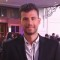Home » Core Java » util » Vector » Set Vector size exampleIlias is a software developer turned online entrepreneur. He is co-founder and Executive Editor at Java Code Geeks.

# Set Vector size example

With this example we are going to demonstrate how to set the Vector size, that is setting a greater or less size than the current size of the Vector. In short, to set the Vector size you should:

• Create a new Vector.
• Populate the vector with elements, with `add(E e)` API method of Vector.
• Invoke the `setSize(int newSize)` API method of Vector. If the `newSize` is less than the current size of the Vector elements after `newSize` index are discarded. If the `newSize` is greater than the current size of Vector, null values are added at the end of the Vector.

Let’s take a look at the code snippet that follows:

```package com.javacodegeeks.snippets.core;

import java.util.Vector;

public class SetVectorSizeExample {

public static void main(String[] args) {

// Create a Vector and populate it with elements
Vector vector = new Vector();

/*

void setSize(int newSize) sets the size of the Vector. If the newSize is less

than the current size of the Vector elements after newSize index are discarded.

If the newSize is grater than the current size of Vector, null values are added

at the end of the Vector.
*/
vector.setSize(3);

System.out.println("Vector(" + vector.size() + ") contains elements : " + vector);

vector.setSize(5);

System.out.println("Vector(" + vector.size() + ") contains elements : " + vector);

}
}
```

Output:

``````Vector(3) contains elements : [element_1, element_2, element_3]
Vector(5) contains elements : [element_1, element_2, element_3, null, null]```
```

This was an example of how to set the Vector size in Java.

# Do you want to know how to develop your skillset to become a Java Rockstar?

## Subscribe to our newsletter to start Rocking right now!

### and many more ....